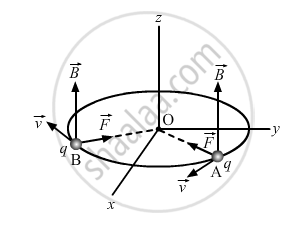# Deduce an Expression for the Frequency of Revolution of a Charged Particle in a Magnetic Field - Physics

Deduce an expression for the frequency of revolution of a charged particle in a magnetic field and show that it is independent of velocity or energy of the particle.

#### Solution

When a charged particle with charge q moves inside a magnetic field vecBwith velocity v, it experiences a force, which is given by:

vecF=q(vecvxxvecB)

Here, vecv is perpendicular to vecB,vecFis the force on the charged particle which acts as the centripetal force and makes it move along a circular path.Let m be the mass of the charged particle and r be the radius of the circular path.

:.q(vecvxxvecB)=(mv^2)/r

v and B are at right angles:

:.qvB=(mv^2)/r

r=(mv)/(Bq)

Time period of circular motion of the charged particle can be calculated as shown below:

T=(2pir)/v

=(2pi)/v(mv)/(Bq)

T=(2pim)/(Bq)

∴ Angular frequency is

omega=(2pi)/T

 :.omega=(Bq)/m

Therefore, the frequency of the revolution of the charged particle is independent of the velocity or the energy of the particle.

Concept: Motion in Combined Electric and Magnetic Fields - Cyclotron
Is there an error in this question or solution?